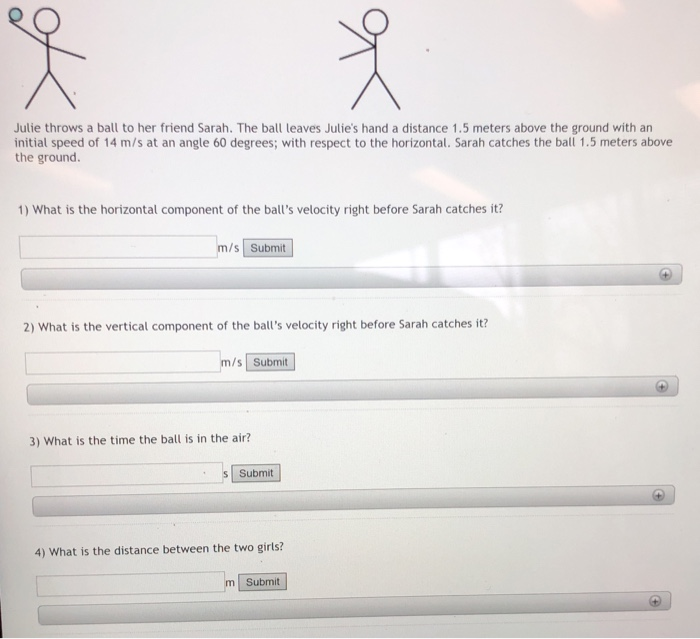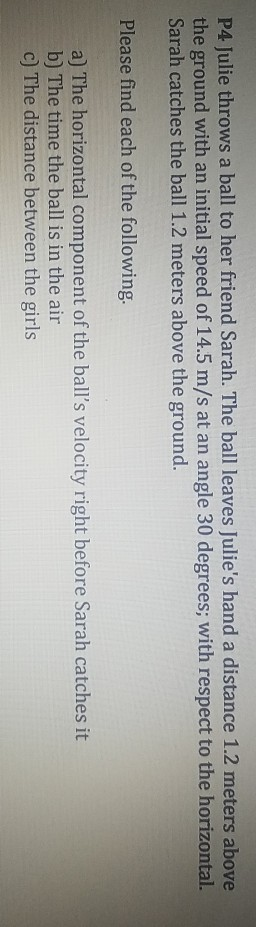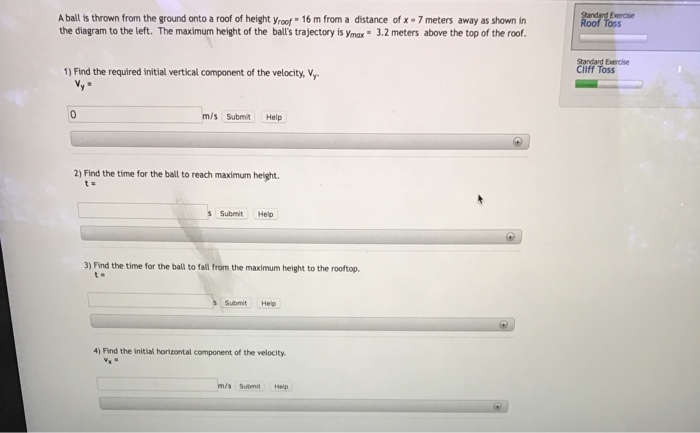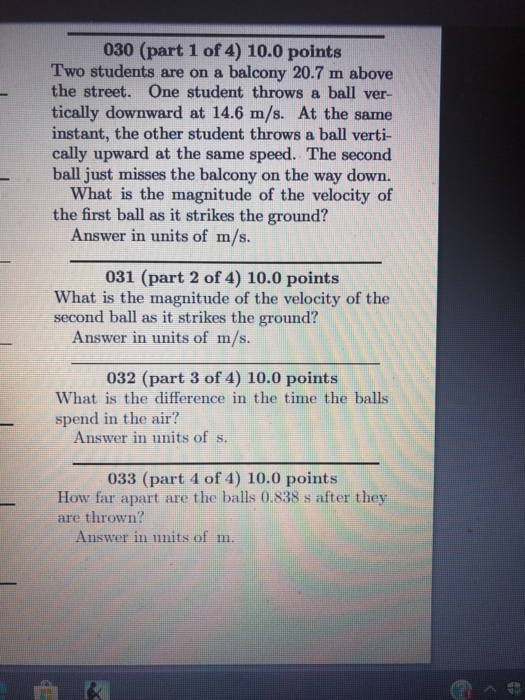Question

# You toss a ball from a balcony. When the ball leaves your hand, it is 10.0...

You toss a ball from a balcony. When the ball leaves your hand, it is 10.0 meters above the ground and has a velocity of (10.0 m/s, 45.0 degrees above the horizontal). Ignore air resistance. (let's take the x-direction to be aligned with the horizontal motion and the y-axis to be upward.)

A) How high above the ground does the ball go, and how much time does it take to reach max height?

B) Determine the total time of flight.

C) How far does the ball land from the base of the balcony?

D) What is the ball's impact velocity?

E) Find the direction of the velocity vector at the point of landing.

a)

height above the ground

H = 10 + (10 sin 45)^2 / (2* 9.8)

H = 12.55 m

time taken to reach the max height

t = 10 sin 45 / 9.8 = 0.7215 s

======

b)

using 2nd equation of motion

0 = 10 + 10 sin 45 * t - 0.5 * 9.8* t^2

solving for t

t = 2.3218 s

=====

c)

x = 10 cos 45 * 2.3218 = 16.417 m

=====

d)

v^2 = 10^2 + 2 * 9.8* 10

v = 17.2 m/s

=====

e)

direction,

cos x = Vx / V

x = arccos ( 10 cos 45 / 17.2)

x = 65.726

======

Comment before rate in case any doubt, will reply for sure.. goodluck

#### Earn Coins

Coins can be redeemed for fabulous gifts.

Similar Homework Help Questions
• ### You toss a ball from your window 14 meters above the ground. When the ball leaves...

You toss a ball from your window 14 meters above the ground. When the ball leaves your hand, it is moving at a speed of 20 m/s at an angle of 10 degree below the horizontal. Choosing a coordinate system such that its origin is located on your hand, the positive x axis is down and the positive y axis is right. Provide all the simplified equations of motion in both directions for this projectile motion problem.

• ### Julie throws a ball to her friend Sarah. The ball leaves Julie's hand a distance 1.5...Julie throws a ball to her friend Sarah. The ball leaves Julie's hand a distance 1.5 meters above the ground with an initial speed of 14 m/s at an angle 60 degrees; with respect to the horizontal. Sarah catches the ball 1.5 meters above the ground. 1) What is the horizontal component of the ball's velocity right before Sarah catches it? /s Submit 2) What is the vertical component of the ball's velocity right before Sarah catches it? /s Submit...

• ### P4 Julie throws a ball to her friend Sarah. The ball leaves Julie's hand a distance...P4 Julie throws a ball to her friend Sarah. The ball leaves Julie's hand a distance 1.2 meters above the ground with an initial speed of 14.5 m/s at an angle 30 degrees; with respect to the horizontal. Sarah catches the ball 1.2 meters above the ground. Wİ Please find each of the following. a) The horizontal component of the ball's velocity right before Sarah catches it b) The time the ball is in the air c) The distance between...

• ### You throw a ball vertically from an apartment balcony to the ground 13.6 m below. Find...

You throw a ball vertically from an apartment balcony to the ground 13.6 m below. Find the ball's initial velocity if it hits the ground 1.08 s after you release it. magnitude? direction? ---Select--- upward downward

• ### A ball rolls off a platform that is 3 meters above the ground. The ball's horizontal...

A ball rolls off a platform that is 3 meters above the ground. The ball's horizontal velocity as it leaves the platform is 4.6 m/s. (a) How much time does it take for the ball to hit the ground? (See the example box, use g = 10 m/s2.) s (b) How far from the base of the platform does the ball hit the ground? m

• ### A ball is thrown from the ground onto a roof of height yroof 16 m from...A ball is thrown from the ground onto a roof of height yroof 16 m from a distance of x 7 meters away as shown in the diagram to the left. The maximum height of the ball's trajectory is ymax 3.2 meters above the top of the roof. Standard Exercise Roof Toss Standard Exercse Cliff Toss 1) Find the required initial vertical component of the velocity, V vy 0 m/s Submit Help 2) Find the time for the ball to...

• ### Julie throws a ball to her friend Sarah. The ball leaves Julie's hand a distance 1.5 meters above...

Julie throws a ball to her friend Sarah. The ball leaves Julie's hand a distance 1.5 meters above the ground with an initial speed of 23 m/s at an angle 28 degrees; with respect to the horizontal. Sarah catches the ball 1.5 meters above the ground. What is the horizontal component of the ball’s velocity right before Sarah catches it? What is the vertical component of the ball’s velocity right before Sarah catches it? What is the time the ball...

• ### You throw your phone straight out horizontally so that is leaves your hand with a velocity...

You throw your phone straight out horizontally so that is leaves your hand with a velocity of 15.6 m/s forward. When the phone leaves your hand, its vertical height is 1 m above the ground. Once it leaves your hand, it begins to fall while moving forward due to gravity pulling it downward. a. What is the phones initial velocity in the horizontal (forward) direction in the instant it leaves your hand? b. What is the phones initial velocity in...

• ### 030 (part 1 of 4) 10.0 points Two students are on a balcony 20.7 m above...030 (part 1 of 4) 10.0 points Two students are on a balcony 20.7 m above the street. One student throws a ball ver- tically downward at 14.6 m/s. At the same instant, the other student throws a ball verti- cally upward at the same speed. The second ball just misses the balcony on the way down. What is the magnitude of the velocity of the first ball as it strikes the ground? Answer in units of m/s. 031 (part...

• ### You toss a ball into the air at an initial angle of 40∘40∘ above the horizontal....

You toss a ball into the air at an initial angle of 40∘40∘ above the horizontal. At what point in the ball’s trajectory does the ball have the smallest speed? (Neglect any effects due to air resistance). at the highest point in its flight just after it is tossed just before it hits the ground halfway between the ground and the highest point on the fall portion of the trajectory halfway between the ground and the highest point on the...